Next: Electric Scalar Potential Up: Electrostatic Fields Previous: Poisson's Equation

# Coulomb's Law

Coulomb's law is equivalent to the statement that the electric field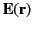generated by a point charge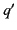located at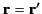is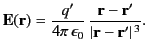(148)

The electric force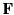exerted on a point charge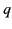located at position vector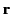is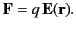(149)

Hence,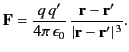(150)

It follows that the electrostatic force acting between two point charges is inverse-square, central, proportional to the product of the charges, and repulsive if both charges are of the same sign.

Electric fields are superposable (see Section 1.2), which means that the electric field generated by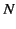point charges,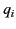, located at position vectors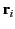, for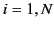, is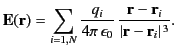(151)

In the continuum limit, the previous expression becomes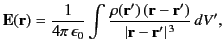(152)

where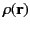is the charge density (i.e., the electric charge per unit volume), and the integral is over all space.Next: Electric Scalar Potential Up: Electrostatic Fields Previous: Poisson's Equation
Richard Fitzpatrick 2014-06-27